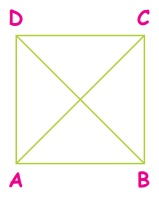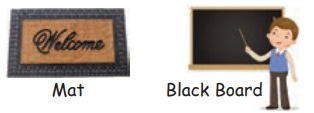Home | | Maths 4th Std | Draw 2D shapes in free hand with geometry tools

# Draw 2D shapes in free hand with geometry tools

Draw 2D shapes in free hand with geometry tools.

1. All closed four sided figures are called quadrilaterals

2. It has four sides (AB,BC,CD,DA), four vertices (A,B,C,D) and two diagonals (AC,BD)EXAMPLES

Square, Rhombus, Rectangle, Trapezium, ParallelogramSquare

A square has four equal sides (PQ=QR=RS=SP), four vertices (P, Q, R, S) and two diagonals (PR, QS).The diagonals of a square are equal in length (PR = QS).

Examples

Carom board, Chess board, One face of the diceRectangle

A rectangle has four sides (WX, XY, YZ, ZW) and four vertices (W, X, Y, Z). It has two equal diagonals (WY = ZX) and opposite sides are equal (WX = YZ ; XY = WZ).Examples

Mat, Black BoardRhombus

A rhombus has four equal sides (LM = MN = NO = OL), four vertices (L, M, N, O) and two diagonals (LN, MO).The diagonals are not equal in length.

ExamplesParallelogram

A Parallelogram has four sides (EF, FG, GH, HE) and four vertices (E, F, G, H). Opposite sides are parallel and equal in length (EF=GH; FG=HE)Activity

Use Geoboard and rubber band to form or deform or reform the different shapes. Discuss similarities and differences among the shapes.

Tags : Geometry | Term 1 Chapter 1 | 4th Maths , 4th Maths : Term 1 Unit 1 : Geometry
Study Material, Lecturing Notes, Assignment, Reference, Wiki description explanation, brief detail
4th Maths : Term 1 Unit 1 : Geometry : Draw 2D shapes in free hand with geometry tools | Geometry | Term 1 Chapter 1 | 4th Maths